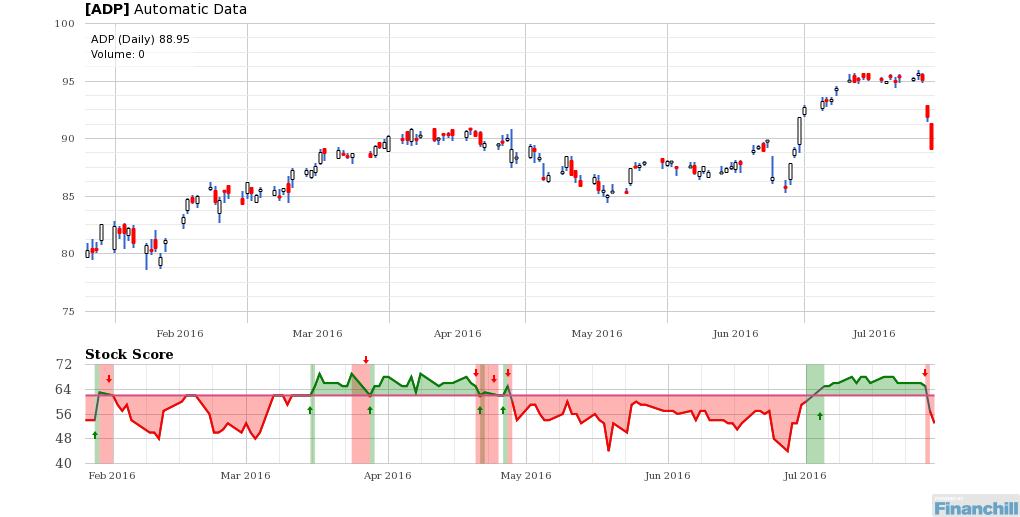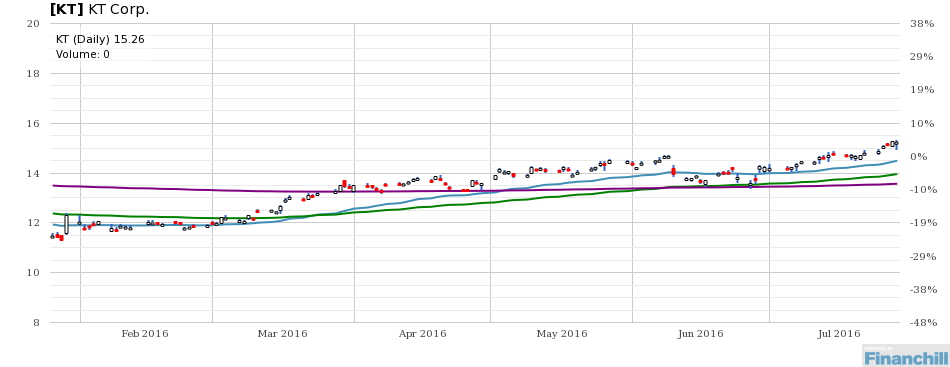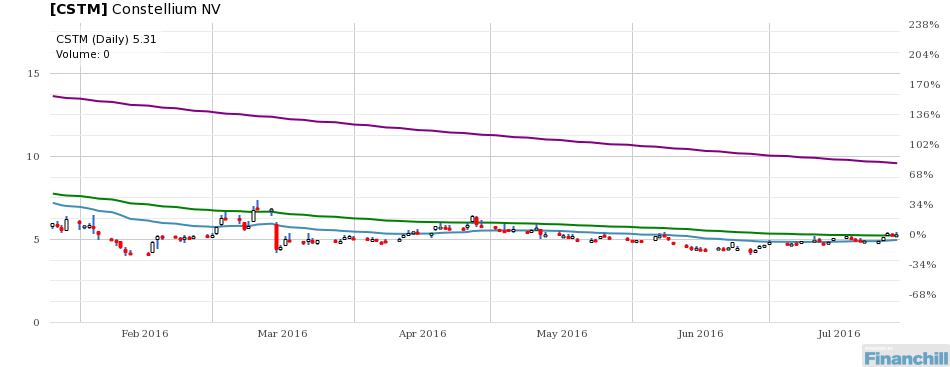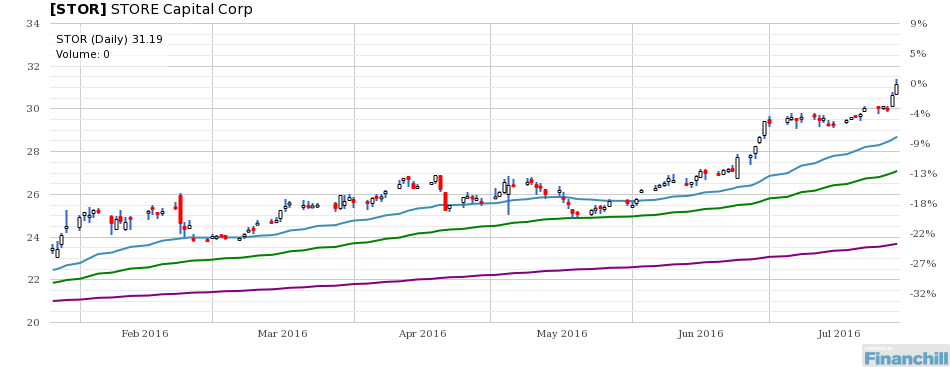# DIN Newsletter for August 1, 2016

## Stock Of The Day: Buy or Sell?

### Automatic Data (ADP) is a SellRisk levels may be somewhat lower than normal.

## CHARTS OF THE DAY

### KT Corp. [KT]

Chart for KTKT share price is \$15.26, which is 5% above its 21-day moving average of \$14.49.

KT share price is \$15.26, which is 9% above its 50-day moving average of \$13.96.

KT share price is \$15.26, which is 12% above its 200-day moving average of \$13.57.

### Constellium NV [CSTM]

Chart for CSTMCSTM share price is \$5.31, which is 7% above its 21-day moving average of \$4.97.

CSTM share price is \$5.31, which is 1% above its 50-day moving average of \$5.24.

CSTM share price is \$5.31, which is 45% below its 200-day moving average of \$9.59.

### STORE Capital Corp [STOR]

Chart for STORSTOR share price is \$31.19, which is 9% above its 21-day moving average of \$28.70.

STOR share price is \$31.19, which is 15% above its 50-day moving average of \$27.10.

STOR share price is \$31.19, which is 32% above its 200-day moving average of \$23.69.

## Economic Indicators

### Total Market Cap / GDP

Chart for Total Market Cap / GDPThe ratio of the Total Market Capitalization (Wilshire 5000) to United States GDP is 1.2204. This is 40.8% above the historic median figure of 0.87.

The higher the Market Cap/GDP figure is relative to its median level, the higher the perceived risk in the market. Markets can remain overvalued for extended time periods.

### S&P Earnings Growth

The S&P Earnings Growth is -12.91. This is 213.6% below the historic median figure of 11.37.

When the S&P Earnings Growth trends lower, the stock market is perceived to have higher risk.

## Sentiment

### VIX

Chart for VIXThe VIX figure is 11.87. This is 33.1% below the historic median figure of 17.74.

The CBOE Volatility Index® (VIX®) is a key measure of market expectations of near-term volatility conveyed by S&P 500 stock index option prices. Historically, VIX levels above 50 correlate closely with periods of stock market panic while VIX levels below 20 are more typical during relatively calm periods in the market.